# Line Graphs

Line Graphs

The world today is becoming more and more information oriented. Every part of our lives utilizes data in one form or another.

The tabular representation of data is an ideal way of presenting them systematically.

When these numerical figures are represented graphically in the form of a bar graph, line graph, etc, or pictorially, they become more noticeable and easily intelligible, leaving a long-lasting effect on the mind of the observer.

In this section, we will learn what line graphs are, its sections, how to read and create graphs and solve interesting problems around it.

Let’s begin our graph of learning!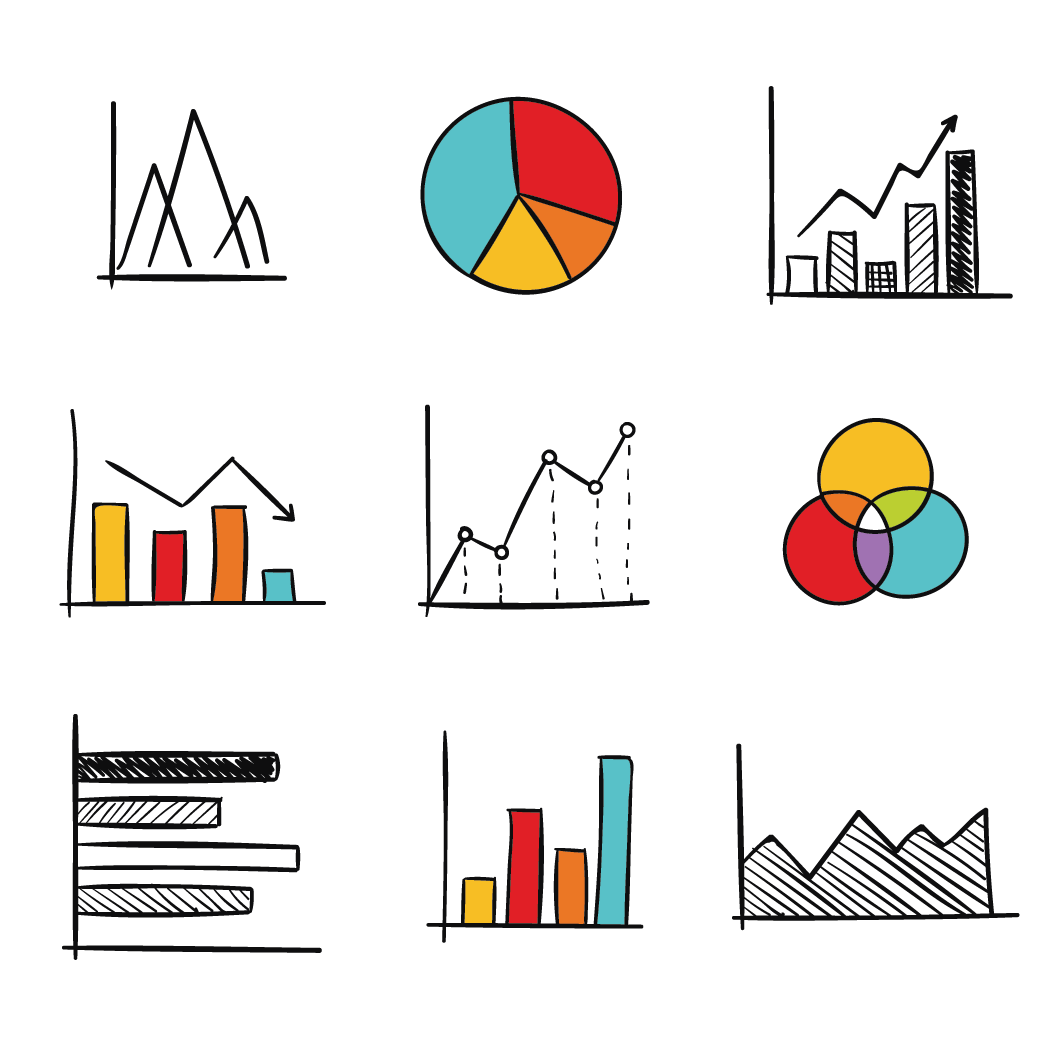## Lesson Plan

 1 What is a Line Graph? 2 Tips and Tricks 3 Solved Examples on Line Graphs 4 Think Out of Box 5 Interactive Questions on Line Graphs

## What is a Line Graph?

Consider the sales report of a company which is represented graphically as below.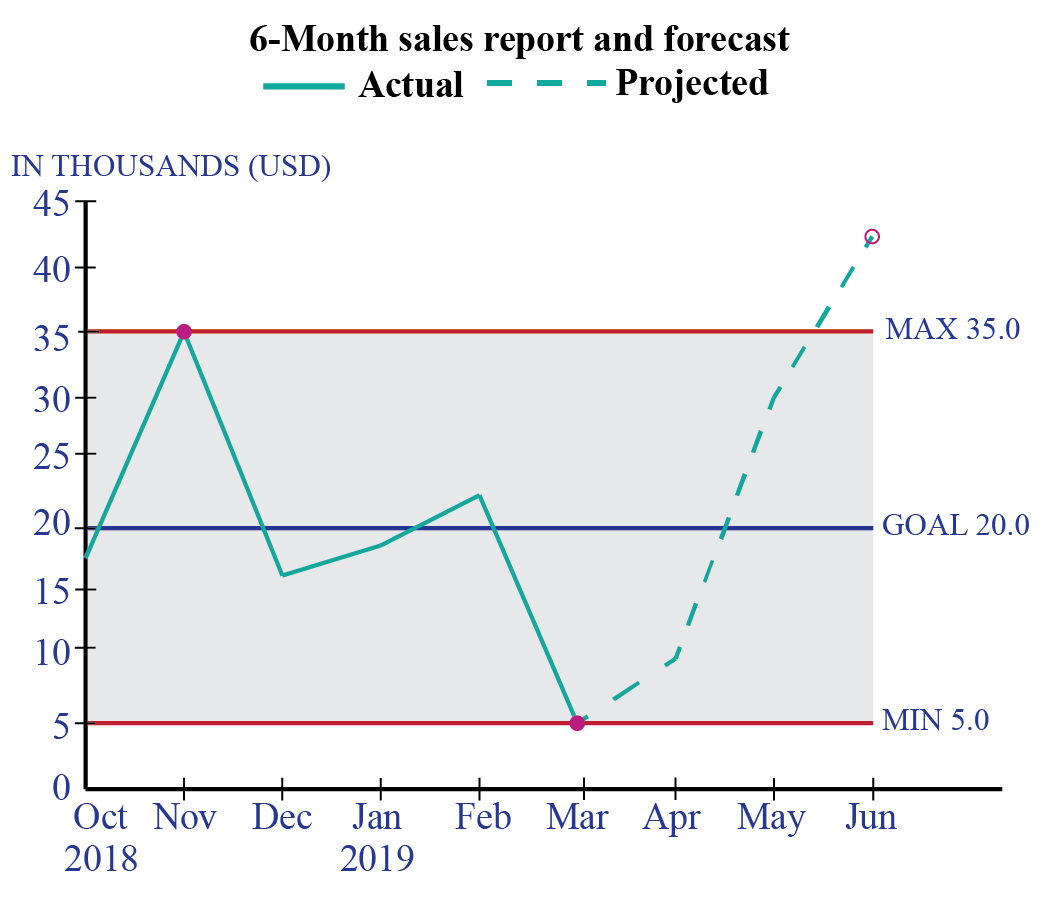Observe carefully and answer these questions.

1. What quantities do $$x$$ and $$y$$ axes represent?

2. Is there use of bars or dots in the graph?

So, we can observe here the graph represents changes in the sales over a time period of 6 months and the expected growth over the next quarter.

Dots are used to show the sales figure in different months and they are joined using line segments. This type of graph is known as a line graph or line chart.

Line graphs are actually cool! They are cool to look at and also if used effectively they can act as powerful tools to increase the efficiency of a task or a function.

The above line graph provides a very good insight into the performance of the company and a deep analysis of the graph helps it to identify effective measures to be taken to improve upon its performance in the coming months.

A line graph is a graph that is used to display change over time as a series of data points connected by straight line segments on two axes.

The line graph also called a line chart. therefore helps to determine the relationship between two sets of values, with one data set always being dependent on the other set.

They are helpful as they demonstrate information factors and patterns unmistakably. Line diagrams can make expectations about the consequences of information not yet recorded.

The slope is the most important observation in this case.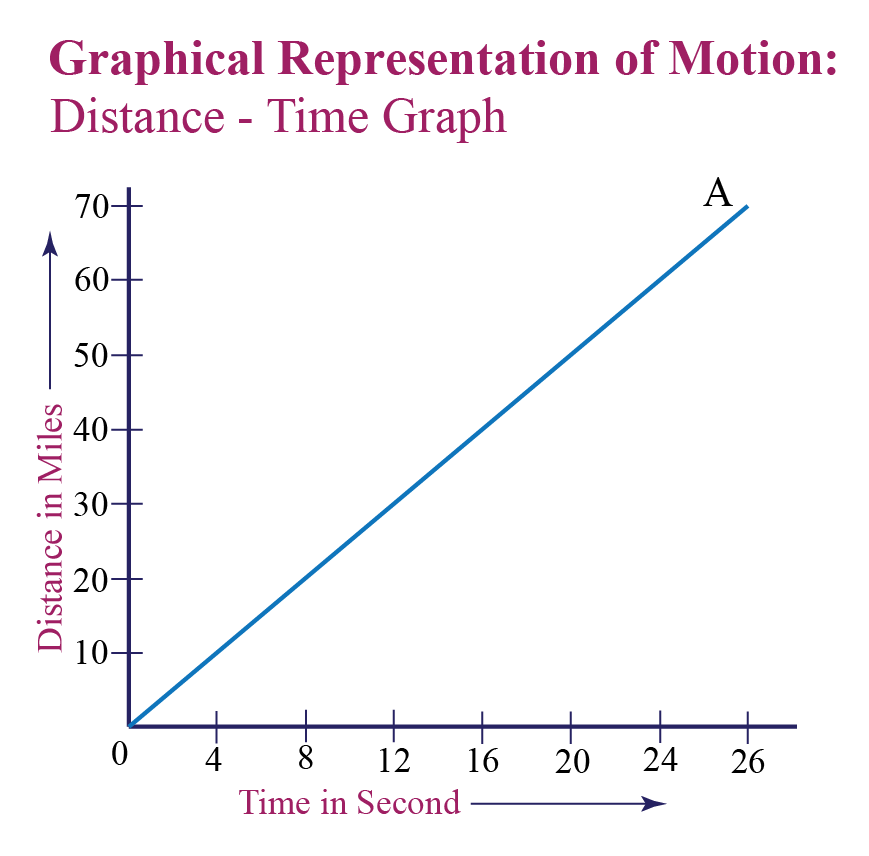It helps in comparing the magnitude of change between any two consecutive points on the graph.

For example: The steeper the slope, the greater is the change in magnitude between two consecutive points.

Look at the distance versus time line graph.

Observe how changing the speed changes the slope of the graph.

## Parts of a Line Graph

The line graph consists of a horizontal x-axis and a vertical y-axis.

Most line graphs only deal with positive number values, so these axes typically intersect near the bottom of the y-axis and the left end of the x-axis.

The point at which the axes intersect is always (0,0).

Each axis is labeled with a data type.

For example, the x-axis could be days, weeks, quarters, or years, while the y-axis shows revenue in dollars.

"Data points are plotted and connected by a line in a "dot-to-dot" fashion."

Let's understand the various parts of a line graph.

### 1.Title

The title of the graph tells us what the graph is all about, i.e what information is depicted by the graph.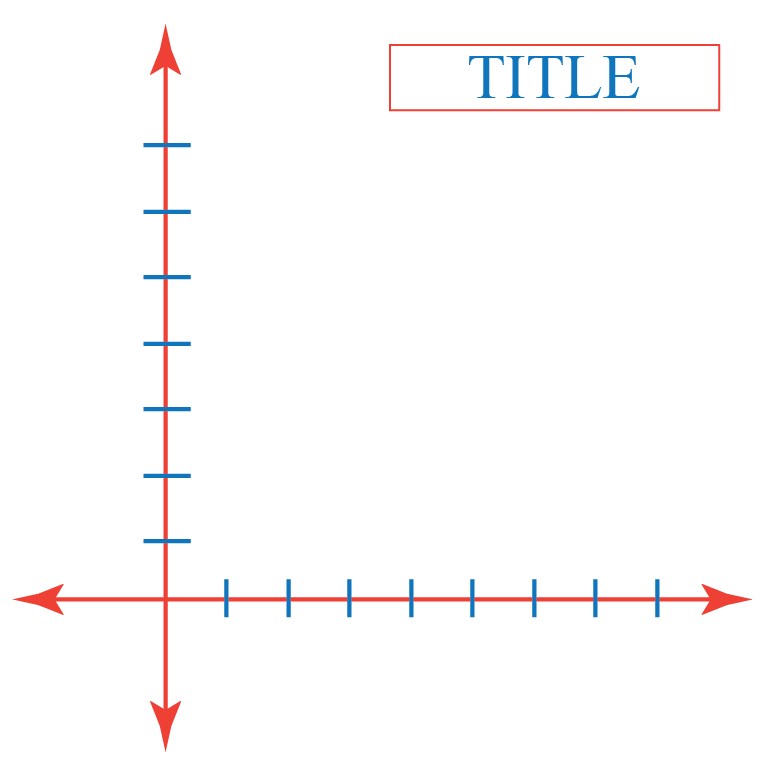### 2.Labels

The horizontal axis across the bottom and the vertical label along the side tell us what kinds of data are being shown.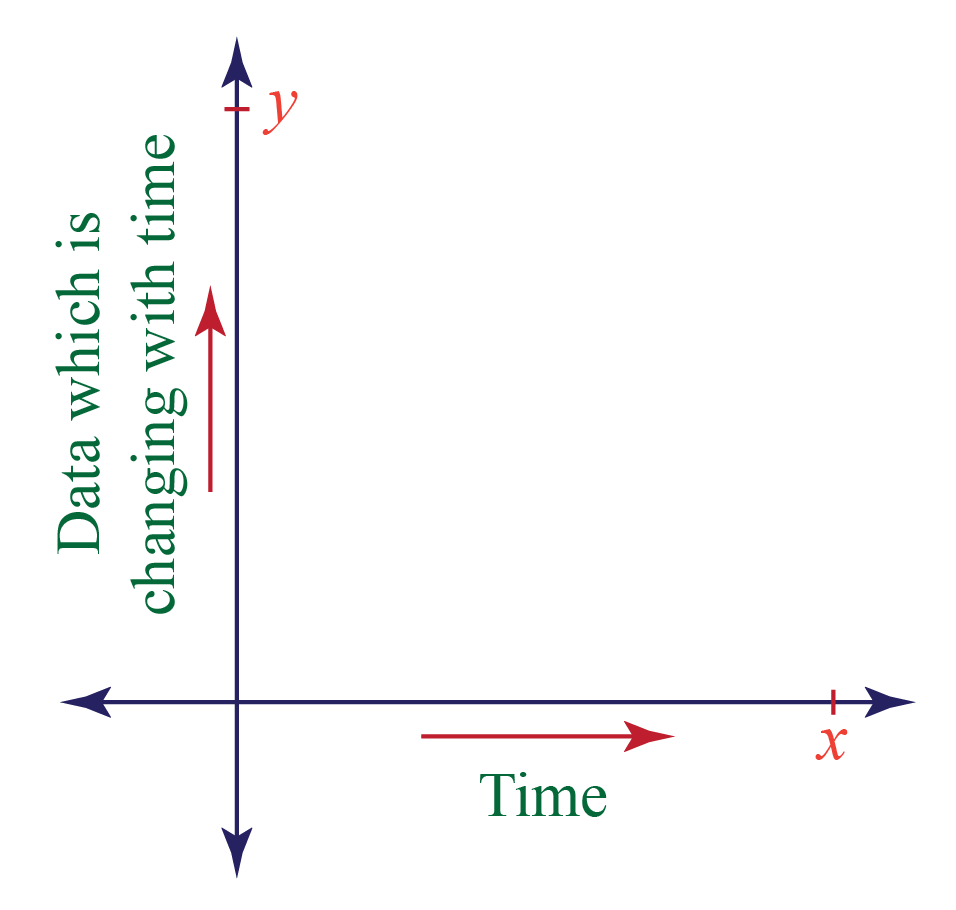### 3.Scales

The horizontal scale across the bottom and the vertical scale along the side tell us how much or how many.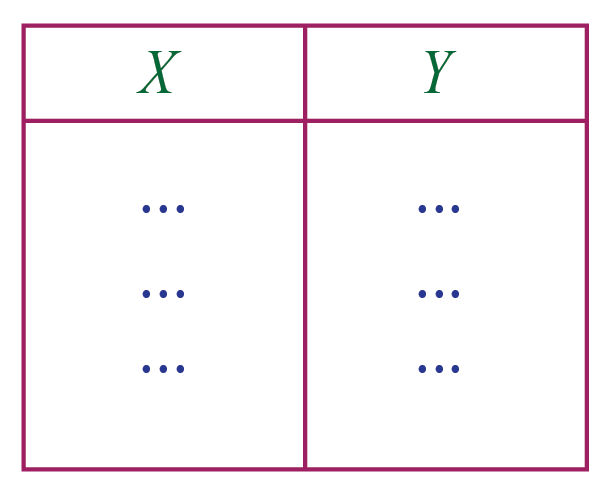### 4.Points

The points or dots on the graph represent the (x,y) coordinates or ordered pairs.

Here data on the horizontal axis is the independent variable and data on the y-axis is the dependent variable.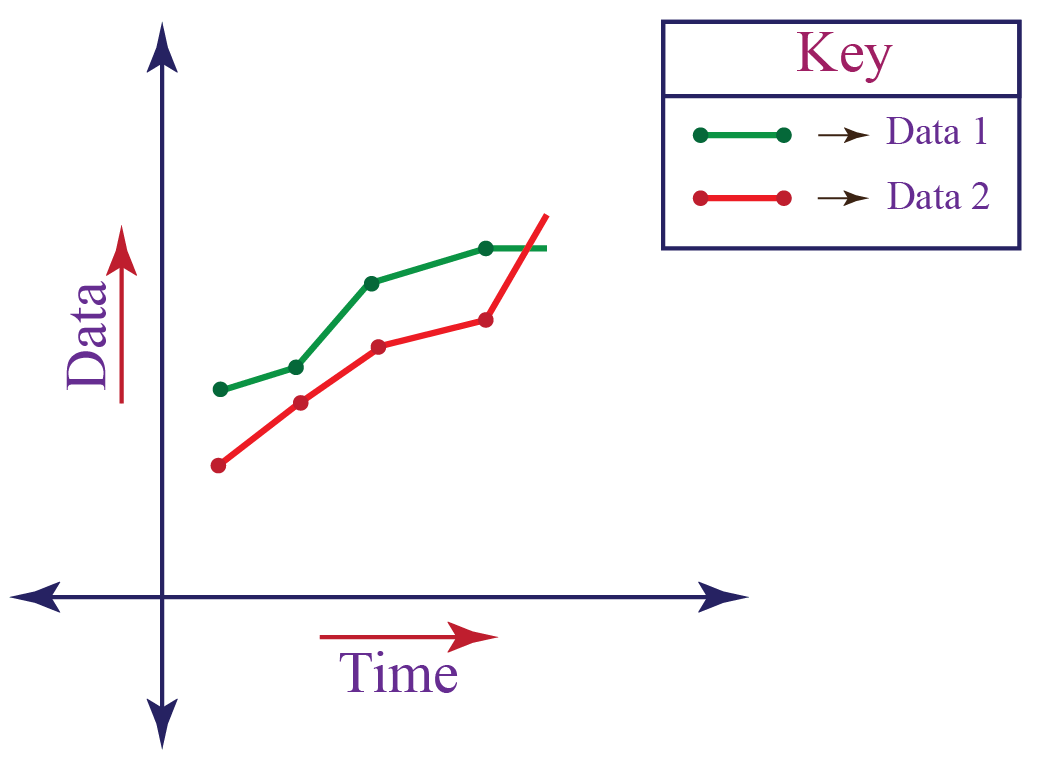### 5.Lines

Straight lines connecting the points give estimated values between the points.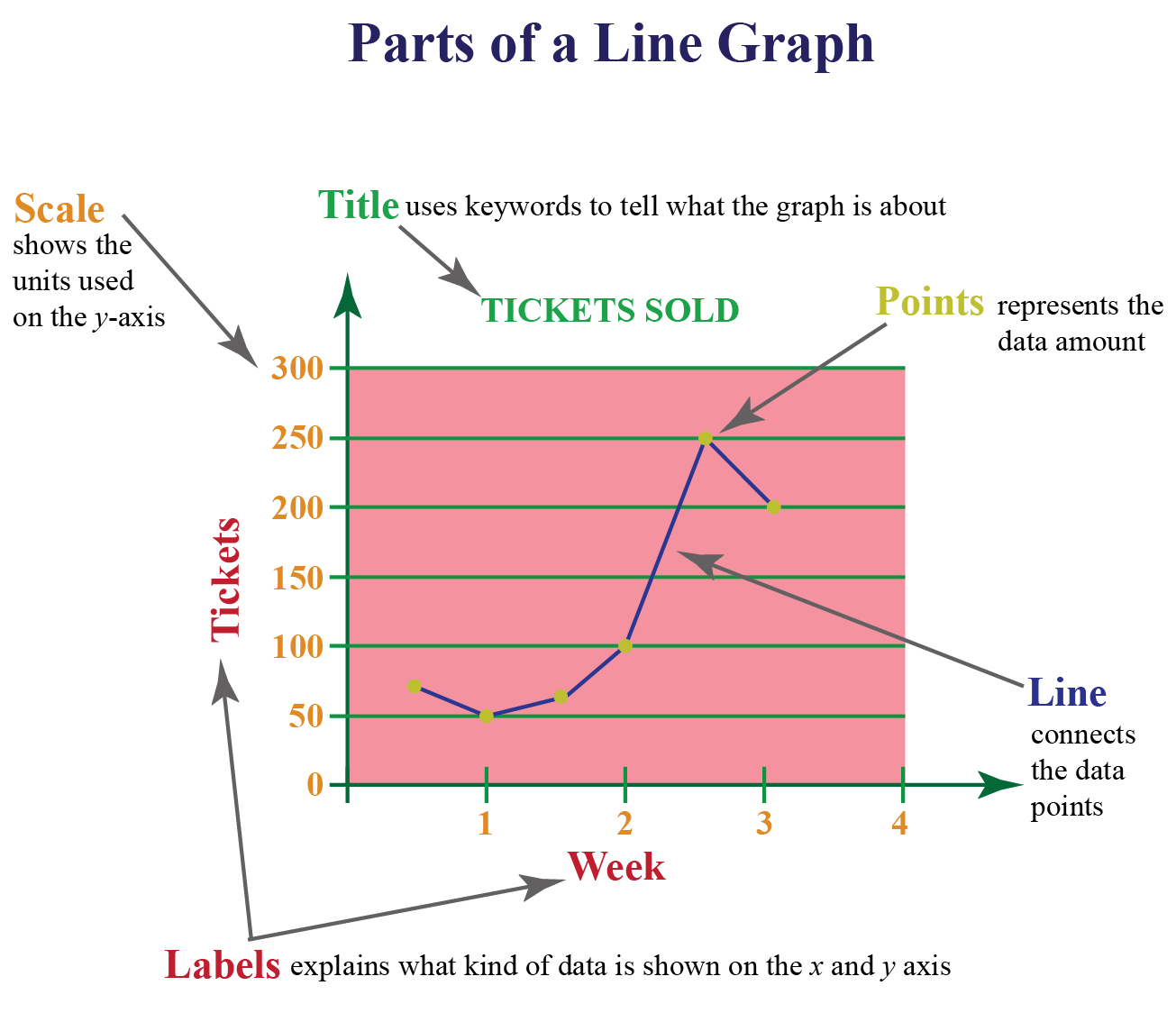While comparing data sets, line graphs are only useful if the x and y axes follow the same scales.

The x-axis is also known as the independent axis because its values do not depend on anything. For example, time is always placed on the x-axis since it continues to change regardless of anything else.

The y-axis is also known as the dependent axis because its values depend on variables on the x-axis: at this time, the company had this much sale.

The result is that the straight line always progresses in a horizontal fashion and for each x value, there is a unique value of y.

E.g. the company cannot have two different value sales at the same time.

## Reading a Line Graph

Consider the following steps to read and interpret a line graph:

• Look at the title.

• See the labeling of axes.

• Check out the emerging patterns to understand the trend.

•  See the data values to get exact figures.

Example

Look at the line graph below and answer the questions that follow.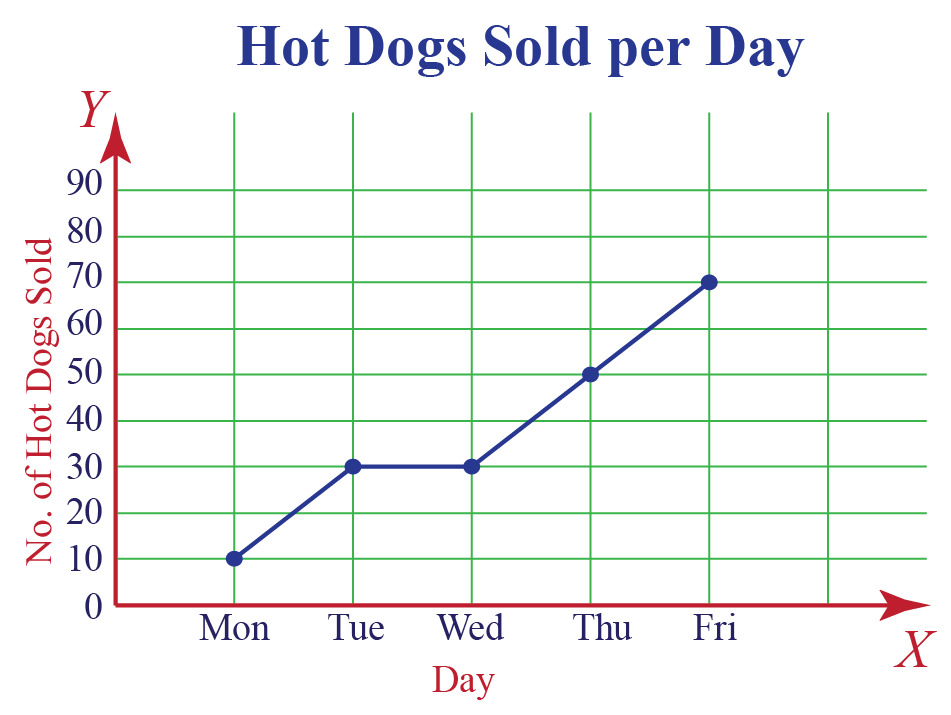1. What information does this graph give?

Solution: This graph provides information about the daily sale of hotdogs on a weekday.

2. How many hotdogs were sold more on Thursday than Monday?

Solution: 50-10=40 were sold more on Thursday than Monday.

3. On which days the sale remains same?

Solution: The sale remains same on Tuesday and Wednesday.

## Creating a Line Graph

Making a line graph is not too hard. Let's understand how to make a line graph taking into consideration the following steps:

1. Create a table

Draw the x- and y-axes on the page. On the top of the page, place a title that briefly describes the purpose of the chart.

2. Label each axis

If time is one of the factors, it should go along the horizontal (x) axis. The other numeric values, i.e the dependent variables measured should be placed along the vertical (y) axis.

Each axis should be labeled with the name of the numeric system as well as the measurements being used.

For example, you may label the x-axis with independent variables like hours or months, indicating that each number written on the axis is thenumber of hours or months.

Divide each axis evenly into applicable increments.

3. Add data

Data for a line graph is usually presented in a two-column table corresponding to the x- and y-axes. Once you've added your data, your line graph will automatically reflect its values.

4. Create a key

If you are comparing multiple items, you'll want to create a key that identifies what each line is by its color.

## Line Graph Simulator

Keeping all the above points in mind, let's try creating one.

Enter the data in the input boxes and see the line graph getting created.

Locate the various components of a line graph in the simulation below.Tips and Tricks
1. Choose the specific title showing exact information.
2. Take variable values in short terms to easily understand.
3. Do not mess with too many lines over the graph. The maximum should be four lines to facilitate comparisons.

### Kinds of Data in a Line Graph:

Line charts can display continuous data over time, set against a common scale, and are therefore ideal for showing trends in data at equal intervals or over time.

As a general rule, use a line chart when your data includes non-numeric (category) data.

For example, a shopkeeper wants to analyze the growth of his business over a period of one year. So, the time factor, i.e. the names of months would be considered on the horizontal x-axis whereas

the vertical y-axis will be used to depict the numerical values, i.e. the monthly profit.

## Advantages of a Line Graph

Look at some benefits of having line graphs.

1. It is beneficial for showing changes and trends over different time periods.

2. It is also helpful to show small changes that are difficult to measure in other graphs.

3. Line graphs are common and effective charts because they are simple, easy to understand, and efficient.

4. It is useful to highlight anomalies within and across data series.

The graph shows the number of items sold by a store in the past 11 days.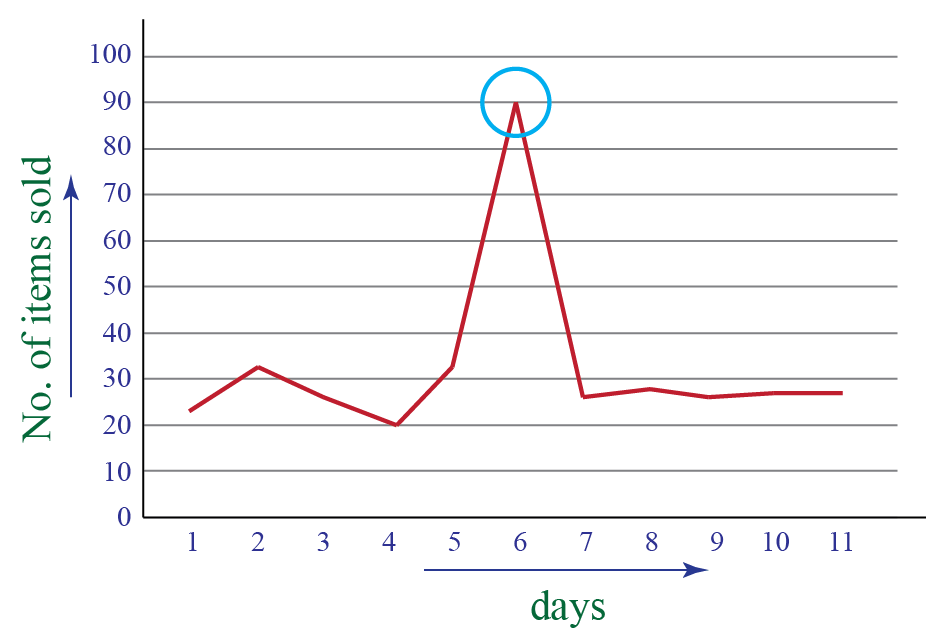There is a  sudden rise on the 6th day showing an increase in sales.

This change in pattern would help the store to identify the sudden increase in the sale and hence plan strategies to increase their sale in the future.

5. More than one line may be plotted in the same axis as a form of comparison.

For example, you could create a line graph comparing the amount of money held by each branch office with a separate line for each office.

In this case, each line would have a different color, identified in a legend.

For example, we could create a line graph comparing the sale of laptop, desktop and tablet over the given time period.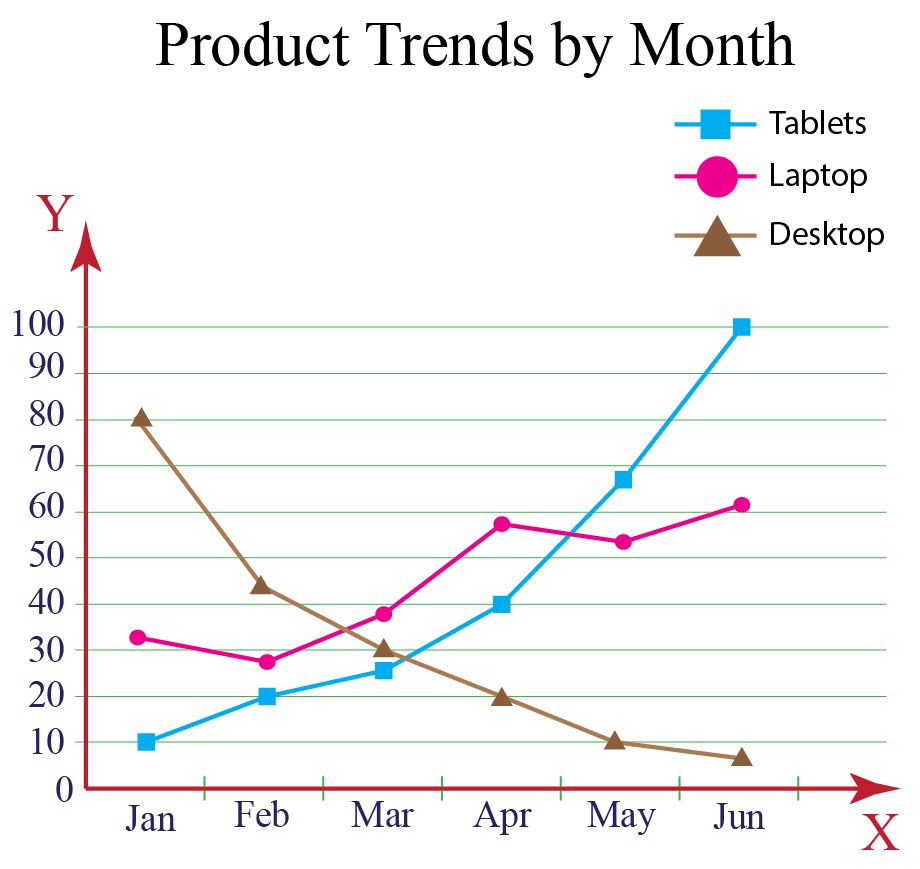## Disadvantages of a Line Graph

Here are some limitations of using line graphs.

1. Plotting too many lines over the graph makes it cluttered and confusing to read.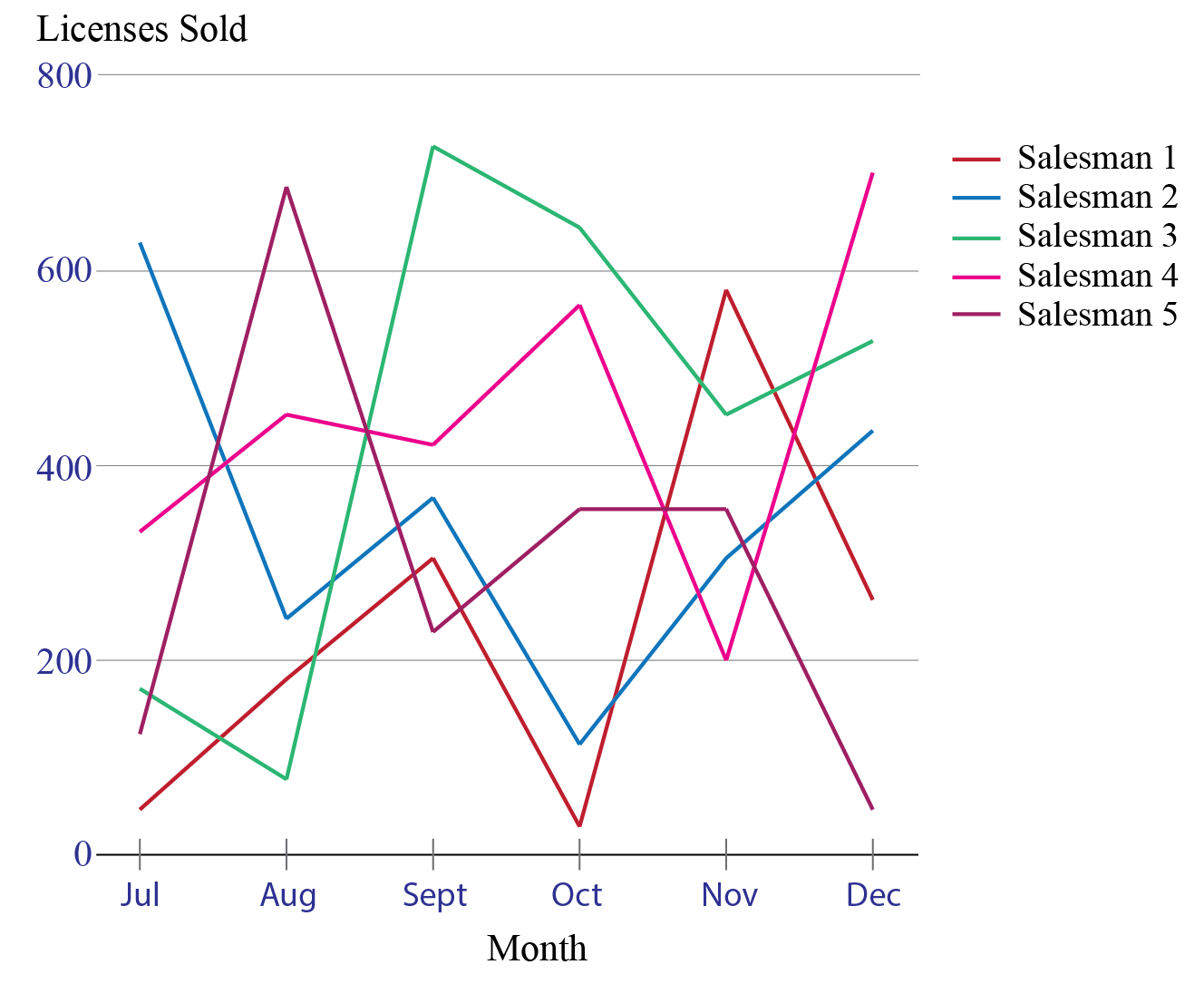2. A wide range of data is challenging to plot over a line graph.

3.They are only ideal for representing data that have numerical values and total figures such as values of total rainfall in a month.

4. If consistent scales on the axis aren't used, it might lead to the data of a line graph appearing inaccurate.

5. Also, line graphs are inconvenient if you have to plot fractions or decimal numbers.

## Scatter Plot Graphs vs Line Graphs

Observe the two graphs shown below and notice the difference between the two,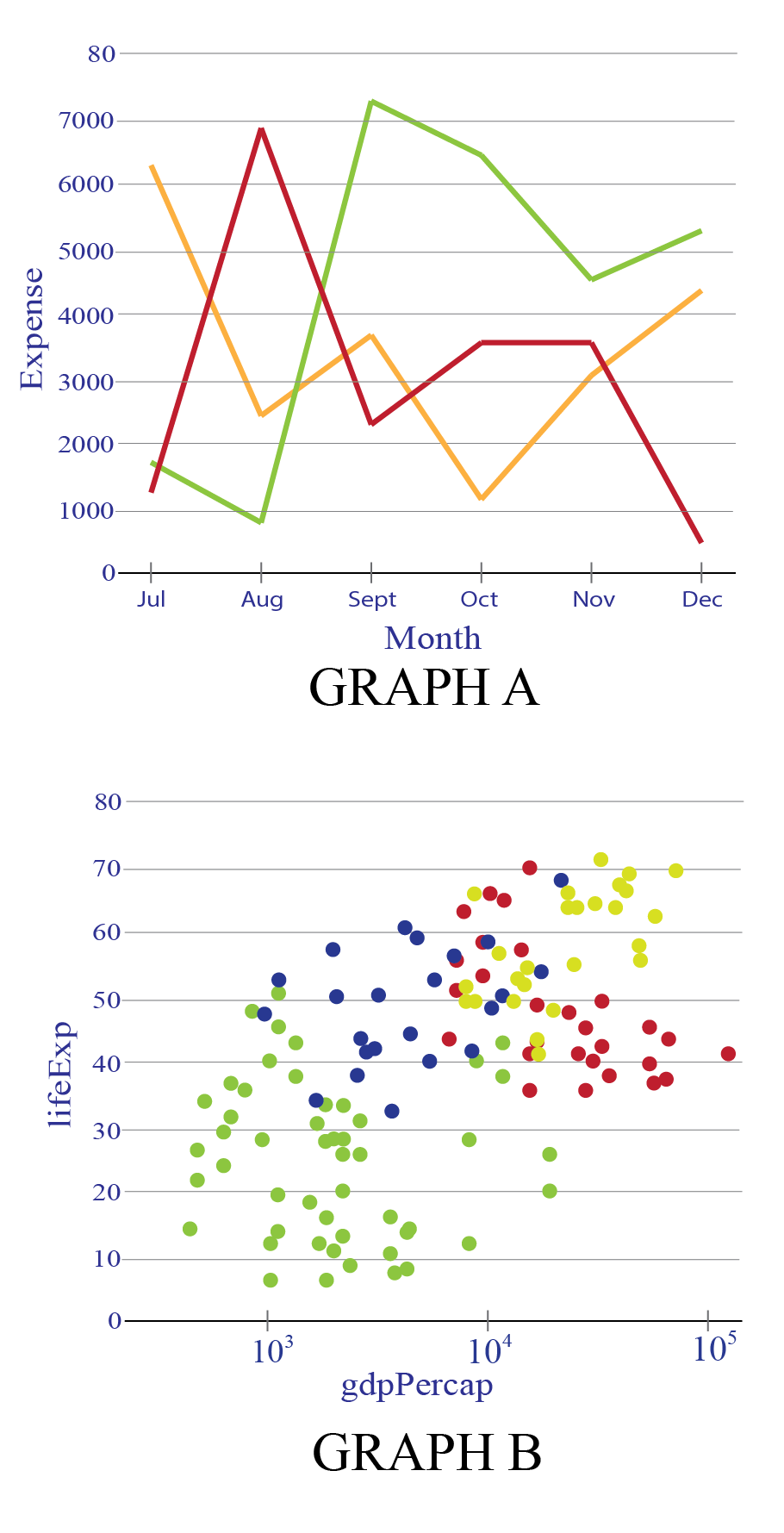Graph A is a line graph whereas Graph B is called the scattered graph or scatter plots.

Line charts and scatter plots are both very helpful and similar for uses.

Choosing the wrong chart type for your data can easily happen when it comes to the line and scatter charts.

These two types of charts record data information on both X and Y axes.

However, it is not easy to select between line and scatter charts since they are very similar, especially when scatter charts are showing connecting lines.

They look very similar, especially when a scatter chart is displayed with connecting lines, but there is a big difference in the way each of these chart types plots data along the horizontal and vertical axes.

 Line graph Scattered Graph Purpose ►Mainly for showing continuous data over a period, setting against a general scale and connecting individual data points together, ideal for showing growth rate or trends at even intervals. ►It works best when comparing large numbers of data points without regard to time. This is a very powerful ►type of chart and good when we are trying to show the relationship between two variables (x and y-axis), for example, a person's weight and height. Axes ►The X-axis can only show texts, which usually refer to non-mathematical data, at equally spaced intervals. ►Data are not evenly distributed. Plotting ►Line segments are used to join dots. ►Dots are not joined. Amount of data ►It can't handle a large amount of data. ►It manages a large amount of data to help in understanding patterns in the data.Think Tank

Which type of graph would be the most suitable to represent the following situations:

1. Percentage fat vs BMI
2. The temperature of the hot liquid as it cools
3. Height of students in a class

## Solved Examples

 Example 1

A bookshop made a line graph of the number of books it sold each week during a certain period.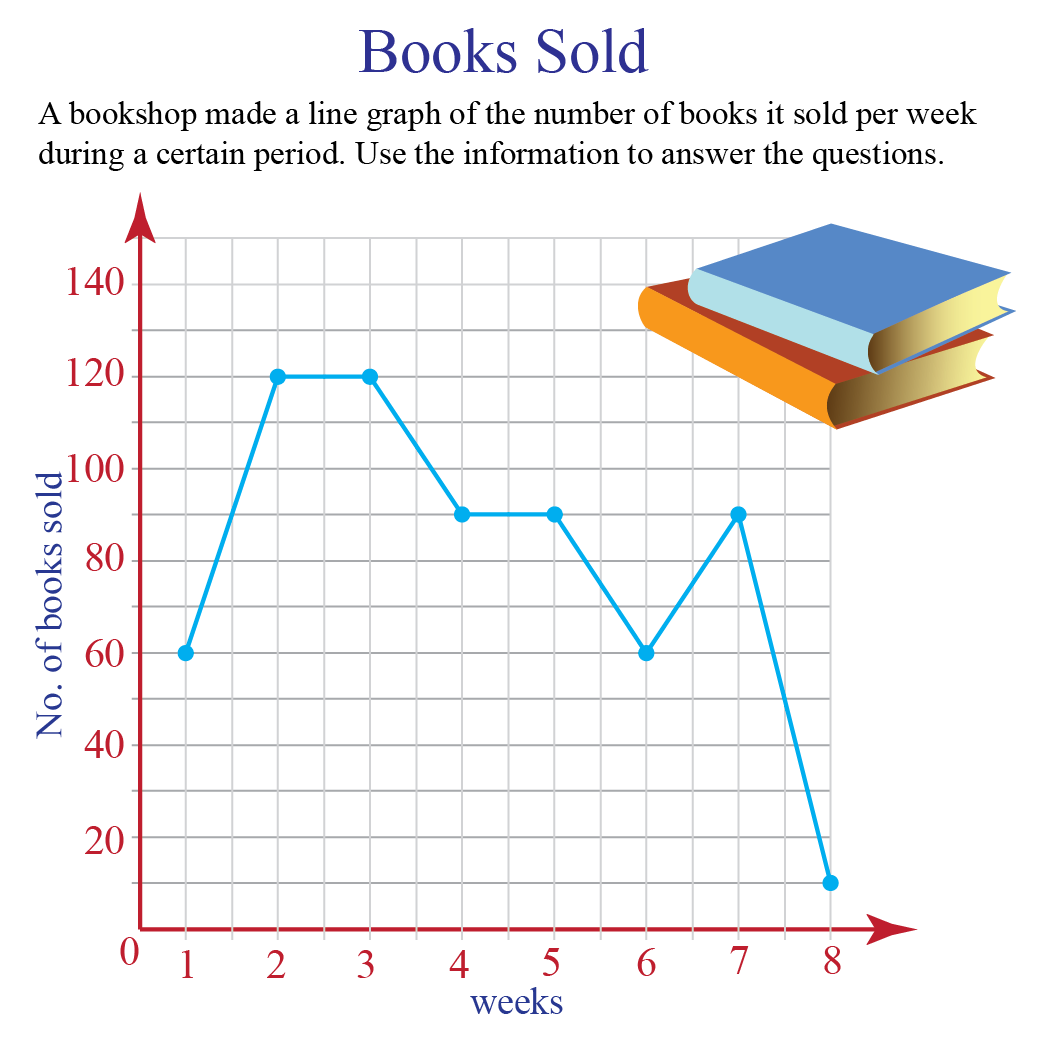Based on the information provided in the above line graph, find how many fewer books were sold in week 8 than in week 7
Solution

No. of books sold in week 7= 90
No. of books sold in week 8= 10
So, difference= 80

 $$\therefore$$ There were 80 fewer books sold in week 8 compared to week 7
 Example 2

The temperature of a city from 5 am to 8 pm on a day was recorded in the form of a line graph as shown below.

Study the graph and answer the following questions.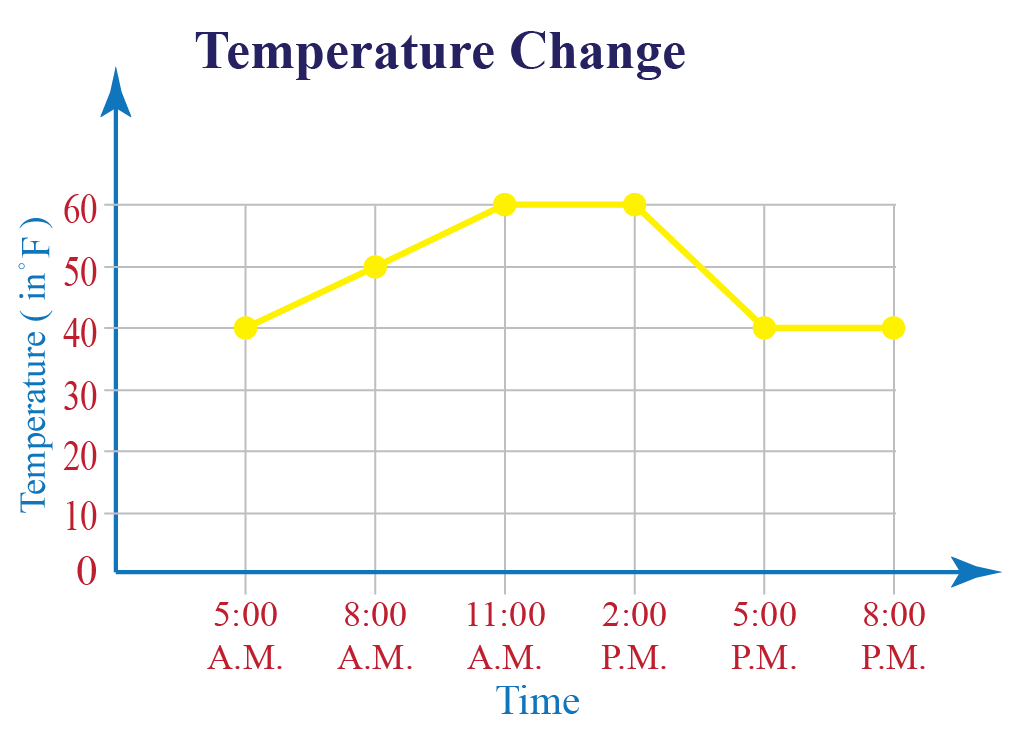Q1: On which day(s) was the temperature 400 F?

Solution

The temperature was 400 F at 5 am and from 5 pm to 8 pm.

Q2: What was the maximum recorded temperature?

Solution

The maximum recorded temperature was 600 F.

 Example 3

Study the following graph to answer the given questions:
Production of two companies A & B over the years are as shown below: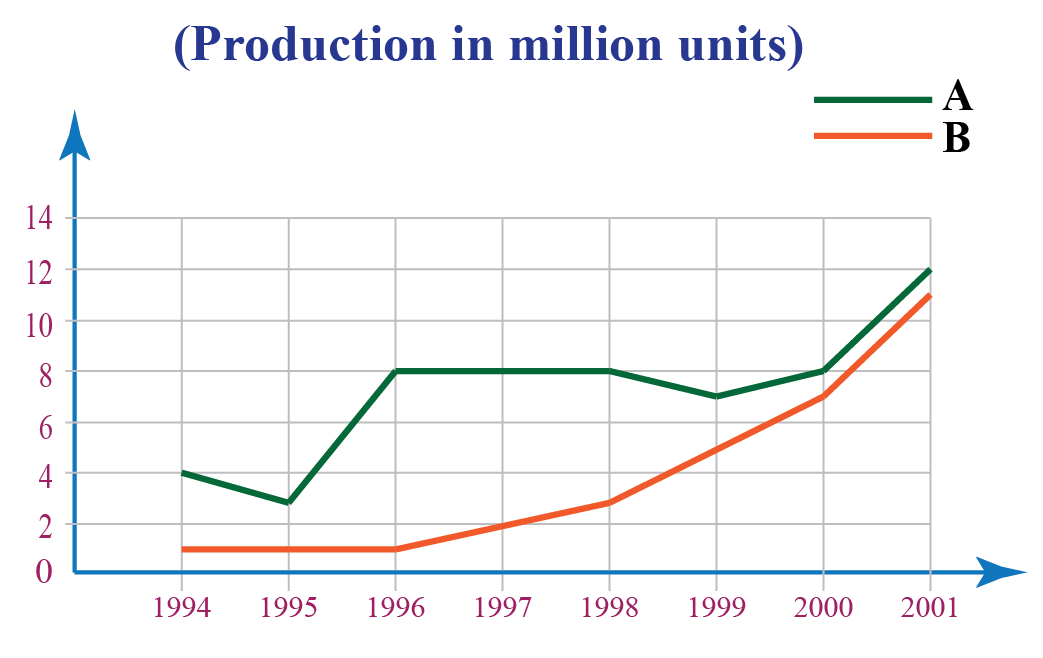For company A, what is the percent decrease in production from 1994 to 1995?

Solution

Difference in production for company A from 1994 to 1995( in millions)$$=4-3$$

Using the formula :

\begin{align} \!\text{% decrease} \!&=\!\!\dfrac{\text{Decrease in production }}{\text{Original production }} \!\!\times\!\! 100\% \\&=\!\dfrac{4-3}{4}\! \times 100 \end{align}

Required percentage decrease = $$\dfrac{1}{4} \times 100 = 25\%$$

 There was a decrease of 25 $$\%$$

## Interactive Questions

Here are a few activities for you to practice. Select/Type your answer and click the "Check Answer" button to see the result.

## Let's Summarize

We hope you enjoyed learning about the Line Graph with the simulations and practice questions. Now you will be able to easily solve problems on a line chart, data points, x-axis, y-axis, line chart, data values, time periods, horizontal axis, vertical y-axis, straight lines, scatter plots, bar graph, independent variable, dependent variable, series of data points, numeric values, and type of graph.

## About Cuemath

At Cuemath, our team of math experts is dedicated to making learning fun for our favorite readers, the students!

Through an interactive and engaging learning-teaching-learning approach, the teachers explore all angles of a topic.

Be it worksheets, online classes, doubt sessions, or any other form of relation, it’s the logical thinking and smart learning approach that we, at Cuemath, believe in.

## 1. What is a straight-line graph?

A straight-line graph is a linear graph involving two variables.

Its general form is : y = mx + c.

where x and y are the variables, m is the slope pf graph and c is constant which is the value of y when x = 0.

## 2. What is a double line graph?

It is a line graph representation of two sets of information on a single graph.

It is generally used for the purpose of comparing the outcomes, information, or events.

Usually, the lines are denoted by different colors to have clarity of the data.

## 3. How many different lines can I put in one chart?

You can put more than one line in a line chart as it helps to compare the data.

It is advisable to use different colors to avoid confusion and errors in interpretation.

Avoid using more than 4-5 lines simultaneously as the lines may become an unreadable tangle and you may have a hard time interpreting them.

More Important Topics
Numbers
Algebra
Geometry
Measurement
Money
Data
Trigonometry
Calculus
More Important Topics
Numbers
Algebra
Geometry
Measurement
Money
Data
Trigonometry
Calculus
Learn from the best math teachers and top your exams

• Live one on one classroom and doubt clearing
• Practice worksheets in and after class for conceptual clarity
• Personalized curriculum to keep up with school